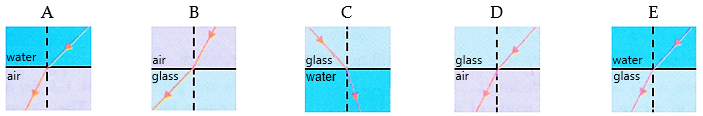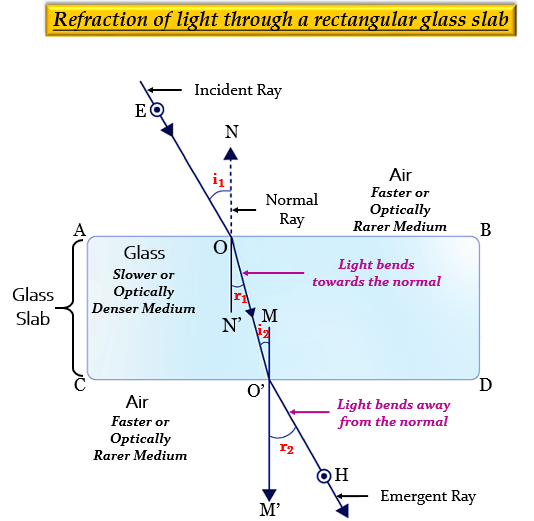"
">

# Which of the following diagrams shows the ray of light refracted correctly?"

Diagram E shows that the ray of light refracted correctly.

Explanation

In diagram A, the light is going from water (denser medium) to air (rarer medium), so it should bend away from the normal, but it bends towards the normal. Hence, it is incorrect.

In diagram B, the light is going from air (rarer medium) to glass (denser medium), so it should bend towards the normal, but it bends away from the normal. Hence, it is incorrect.

In diagram C, the light is going from glass (denser medium) to water (rarer medium), so it should bend away from the normal, but it bends towards the normal. Hence, it is incorrect.

In diagram D, the light is going from glass (denser medium) to air (rarer medium), so it should bend away from the normal, but it bends towards the normal. Hence, it is incorrect.

In diagram E, the light is going from water (rarer medium) to glass (denser medium), so it should bend towards the normal, and this principle is followed by this diagram. Hence, it is correct.Updated on: 10-Oct-2022

92 Views